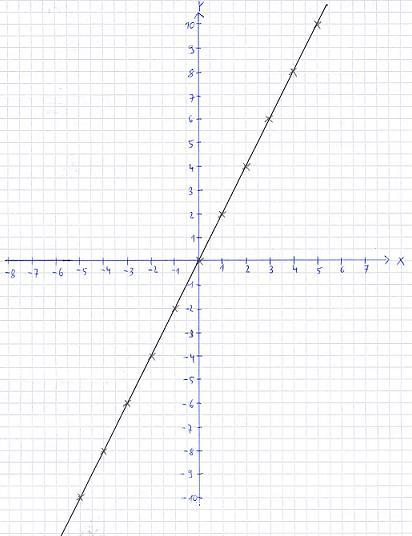# How many lines go through two points

## Linear function through 2 pointsSometimes you look for a role in a math class. Then different points are given and a function is to be determined that runs exactly through these points. To understand the next few sections you should know what a linear function is and how to solve a linear system of equations.

Show:

### Even / linear function through 2 points

A straight line or a linear function is described with f (x) = y = mx + b. The variables m and b are unknown. To determine this we need two points. With this we set up two equations and solve the linear system of equations to determine m and b. This will now be shown by means of examples.

Example 1:

On the following graphic you can see a linear function. We are looking for a mathematical description of this process. To do this, we first read two points on the graphic. This would be for example P1 (0 | 0) and P2 (1 | 2). So we have two points, each with an X value and a Y value. We put both points in y = mx + b. We thus get two equations:

• P1: 0 = m * 0 + b
• P2: 2 = m * 1 + b

From the first equation we see that b = 0. If we plug this into the second equation, we still get m = 2. So after plugging in y = mx + b we get:Example 2:

There are two points with P1 (2 | 3) and P2 (5 | 6). What is the corresponding linear function?

Solution: We insert both points in y = mx + b and then solve the linear system of equations.

• P1: 3 = m * 2 + b
• P2: 6 = m * 5 + b

We convert the first equation to b:

And plug this into the second equation:

• 6 = 5m + b
• 6 = 5m + 3 - 2m
• 3 = 3m
• m = 1

With m = 1 we go into the first equation:

• 3 = 2m + b
• 3 = 2 x 1 + b
• 3 = 2 + b
• b = 1

With b = 1 and m = 1 we get:

Left:

### Who's Online

We have 893 guests online